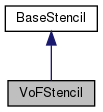Chombo + EB  3.0
VoFStencil Class Reference

VoF-centered stencil. More...

#include <Stencils.H>

Inheritance diagram for VoFStencil:[legend]

Public Member Functions

VoFStencil ()

virtual ~VoFStencil ()

void clear ()

void add (const VolIndex &vof, const Real &weight, int ivar=0)

virtual int size () const

const VolIndexvof (int i) const

virtual BaseIndexindex (int i) const

virtual const Realweight (int i) const

virtual Realweight (int i)

virtual const int & variable (int i) const

virtual int & variable (int i)

VoFStenciloperator+= (const VoFStencil &a_vofsten)

void operator*= (const Real &scaling)

VoFStenciloperator= (const VoFStencil &a_vofsten)

VoFStencil (const VoFStencil &a_vofstenin)

void setAllVariables (int a_var)Public Member Functions inherited from BaseStencil
BaseStencil ()

virtual ~BaseStencil ()

Protected Attributes

Vector< VolIndexvofs
the VoFs More...

Vector< Realweights
the weights More...

Vector< int > variables

Detailed Description

VoF-centered stencil.

This stencil is a fundamental tool for building eb applications by making the ability to cache stencil information. This object consists of a vector of VoFs and a vector of weights.

Constructor & Destructor Documentation

 VoFStencil::VoFStencil ( )

default constructor. makes empty vectors.

 virtual VoFStencil::~VoFStencil ( )
virtual
 VoFStencil::VoFStencil ( const VoFStencil & a_vofstenin )

Member Function Documentation

 void VoFStencil::clear ( )
 void VoFStencil::add ( const VolIndex & vof, const Real & weight, int ivar = 0 )

add a VoF to the Stencil, with it's associated weight it is required that all VoFs are in the same EBIndexSpace If the vof is already in the stencil, add the weights.

 int VoFStencil::size ( ) const
inlinevirtual

number of VoFs in the Stencil

inlines

Implements BaseStencil.

References Vector< T >::size(), and weights.

 const VolIndex & VoFStencil::vof ( int i ) const
inline

access a VoF

References vofs.

Referenced by index().

 virtual BaseIndex& VoFStencil::index ( int i ) const
inlinevirtual

Implements BaseStencil.

References vof().

 const Real & VoFStencil::weight ( int i ) const
inlinevirtual

access a weight

Implements BaseStencil.

References weights.

 Real & VoFStencil::weight ( int i )
inlinevirtual

access a weight

References weights.

 virtual const int& VoFStencil::variable ( int i ) const
virtual

Implements BaseStencil.

 virtual int& VoFStencil::variable ( int i )
virtual
 VoFStencil& VoFStencil::operator+= ( const VoFStencil & a_vofsten )

add all faces and weights of inputs to this. If a vof is already in the stencil, add the weights. only addition is well-defined here as far as arithmatic operations are concerned.

 void VoFStencil::operator*= ( const Real & scaling )
 VoFStencil& VoFStencil::operator= ( const VoFStencil & a_vofsten )
 void VoFStencil::setAllVariables ( int a_var )
inline

References Vector< T >::size(), variables, and vofs.

Member Data Documentation

 Vector VoFStencil::vofs
protected

the VoFs

Referenced by setAllVariables(), and vof().

 Vector VoFStencil::weights
protected

the weights

Referenced by size(), and weight().

 Vector VoFStencil::variables
protected

Referenced by setAllVariables().

The documentation for this class was generated from the following file: